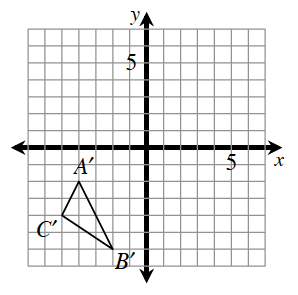### Home > INT2 > Chapter 6 > Lesson 6.2.2 > Problem6-67

6-67.

$ΔABC$ was reflected across the $x$-axis, and then that image was rotated $90^\circ$ clockwise about the origin to result in $ΔA'B'C'$, shown at right. Determine the coordinates of points $A, B,$ and $C$ of the original triangle.Perform the transformations opposite and in reverse.
Rotate the given triangle counterclockwise $90^\circ$ and then reflect it across the $x$-axis.